Physic Tutorials

# Heat Capacity and Specific Heat Capacaity | Introduction

Heat capacity is defined as the heat required to raise the temperature of the body through 1K. The unit is joules per Kelvin (J/K).

Specific heat capacity of a substance is the quantity of heat required to raise the temperature of unit mass (1kg) of the substance by 1K or 1oC. The S.I unit is J/kgk or J/kgC

The law of conservation of energy shows that the heat we supply does not just disappear. It is usually transformed into some other kind of energy. In this case, the heat supplied to the water is transformed to the internal energy of the water molecules. I shall focus on conservation principle of heat energy, H.C, S.H.C, and latent heat.

## Formula for Heat Capacity and Specific Heat Capacity

H.C= mass of substance x S.H.C

S.H.C =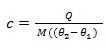From the definition of S.H.C, it follows that

Heat capacity of 5kg of aluminium = Mass (5kg) x S.H.C of aluminium (910 J/kg.k) = 4550 J/K

or

H.C of 2kg of water = mass (2kg) x S.H.C of water (4200 J/kg.k) = 8400 J/K

Factors that does not affect specific heat capacity of a material

• It doesn’t depend on how much of the material is present.
• It’s also independent of the temperature interval i.e. if you double or tripple the teperature S.H.C is not affected

## Specific Heat Capacities for some substances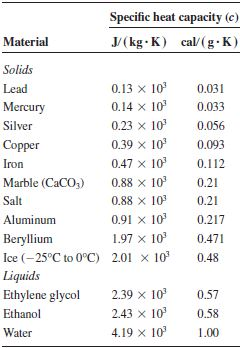Example1 Calculate the quantity of heat required to raise the temperature of 4kg of copper from 250C to 950C [Take S.H.C of copper = 390 J/Kgk]

Quantity of heat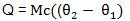M = 4kg, C = 390J/Kgk, (  = 95 – 25 =70

Q = 4 x 390×70

Q = 109200J

Determination of specific heat capacity of a solid

1. Method of Mixture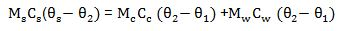2. Electrical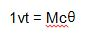Example 2: A piece of copper block of S.H.C 400J/kgk falls through a vertical distance of 20m from rest, calculate the rise in temperature of the copper block on hitting the ground when all its energies are converted into heat.This potential energy is converted into kinetic energy as it falls and the kinetic energy to heat energy as it hits the ground.

Example 3:  A heating coil is rated 75W, calculate the time it will take this coil to heat 1.4kg of water at 300C to 1000C (specific heat capacity of water =4200J/KgK)

Heat energy supplied by the heater = Heat absorbed by water

Ivt = Mcq

But power = IV,

Energy = Pt = Mcq

75 x t = 1.4 x 4200 x (100 – 30)

t = 54885 s

Change of state

Substance can exist in any three state of matter namely solid, liquid or gas. The state in which a substance exists depends on the temperature. Solid when heated change to liquid and when the liquid is further heated, it changes to gas.

Note: during change of state temperature remain constant.

The heat supplied to a solid (e. g ice) during change of state is used to overcome the attractive forces that hold the solid molecule together. It does not make the substance warmer and so it is not detected by a thermometer. The heat is used mainly in making the solid molecule move freely as in a liquid. Also the heats supply to a liquid at its boiling point is used to overcome the attractive forces that hold the liquid molecule together and push back the surrounding air molecule.

Q = ML (Joule)

Where L is called the specific latent heat

L = Q/M = J/kg

Example 4: Calculate the amount of heat energy required to change 20kg of ice water at 00C. Specific content heat of ice = 336 x  103 J/kg

Q = 20 x 366 x

Q= 732 x  J

Q= 7.32 x J